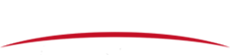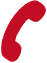﻿ How to Use a Multimeter - Hang Zhou Tronstol technology Co., Ltd.
86-571-26265158The multimeter is often used in the inspection and maintenance of the SMT machines. As its name states, it is a meter capable of measuring multiple things related to electricity, namely: Voltage, Current and Resistance.

## Continuity

Continuity is quite possibly the single most important function for troubleshooting and debugging circuits. This feature allows us to test for conductivity of materials and to trace where electrical connections have been made or not made. Set the multimeter to “Continuity”mode. It may vary among DMMs, but look for a diode symbol with propagation waves around it (like sound coming from a speaker).

Touch the two probe ends together. Do you hear the tone? Checking for continuity is sometimes also called "ringing out" a  circuit. You can use this to test which holes on a breadboard are connected and which ones aren’t. You can also use this to trace out a circuit. Because you often can't see where all of the wires go, this is a quick way to test to see if two points are connected electrically.

## Measuring Resistance

Similar to testing for continuity is measuring resistance. The continuity setting simply rings a tone when the resistance is low (on the order of a few ohms). But, if you want to know an actual value for the resistance, this is the setting you want. Turn the knob to one of the resistance settings. These are marked by the Omega symbol(Ω). This represents the unit ohms which is used to measure resistance.

There are several settings possible. These are ranges and represent the maximum range that you can measure. If you want to measure a small resistor to a high accuracy, you might set the multimeter to the 200Ω setting. Notice that there are no zeros displayed. This means that the meter senses the resistance to be greater than the range. Try moving the range up a notch and measuring it again.

Because the display has no units on it, it is assumed that the number displayed matches the same units as the setting. If you have the range set to 200Ω, then the display will be in Ω. If you have the range set to 2kΩ, 20kΩ, or 200kΩ, then the value displayed will be in units of kΩ.

## Measuring Voltage

Voltage is a measurement of electrical potential between two points. It is sometimes also called the potential difference. Similar to the settings when measuring resistance, the various settings for measuring voltage specify the maximum range.

You will notice that there are two different ranges when measuring voltage. The one on the top has two straight lines and the one below has a squiggly line. The two straight lines indicate direct current(DC) measurements. This is what is most common in electronics. The other one is alternating current(AC)，and this is the type of electricity that is found in the walls of your house. Be sure that you have the knob turned to the right setting. The 20V setting is probably the most typical for our applications.

Now, lets measure the voltage on an Arduino board. Plug your Arduino board into your computer using the USB cable for power. To measure voltage, connect the black probe to GND or ground. Now, use the red probe to test the voltage at various points (with respect to GND).

## Measuring Current

Current is the rate of moving charges in a circuit. We now know that this is the movement of electrons, but by convention, we still look at current as the movement of charges from positive(+)to negative (-). In order to capture the rate of moving charges, you need to break the circuit and put the meter in-line where you want to measure current. Move the knob to the appropriate current range at you expect to measure. If you are measuring anything above 200 MA, you need to also move the red probe to the 10A port.

To measure the current going through a simple LED and resistor circuit, for example, you would have to first splice into the circuit somewhere. Here, we spliced into the circuit just after the LED. The current path must go through the meter. Because this is a series circuit, you can measure the current anywhere along this path.

When you are measuring current, be very careful not to exceed the limits. When you go beyond the current limits, you run the risk of also blowing a fuse on the multimeter. The standard MAvΩ port can handle up to 200 MA. If you think you'll be measuring more than this, move the red probe to the 10A port and change knob to the 10A setting.

Relate News
4th Floor, Building 5, No. 2, Huayi Road, Yuhang Street, Yuhang District, Hangzhou